Published: 31 March 2017

# Bursting oscillation and bifurcation mechanism in fractional-order Brusselator with two different time scales

Jufeng Chen1
Xianghong Li2
Jingyu Hou3
Dawei Zuo4
1, 2, 3, 4Department of Mathematics and Physics, Shijiazhuang Tiedao University, Shijiazhuang, 050043, China
Corresponding Author:
Xianghong Li
Views 387

#### Abstract

The integer-order and fractional-order Brusselators with two different time scales are studied. The double-Hopf bursting oscillation is observed in Brusselator with periodic perturbation under some parameter conditions. Based on slow-fast analysis and bifurcation theory, the generation mechanism of periodic bursting oscillation is presented in detail. Further investigation finds that the perturbation amplitude plays an important role on bursting oscillation. With the decrease of perturbation amplitude, the attractor types will be changed, so that the bi-stability evolves into single stability. It causes that four times transitions between spiking and quiescent states may decrease to twice one, and the spiking state disappears finally. Furthermore, the influence of the fractional order on bursting behavior is investigated, and the bifurcation diagram with respect to fractional order and slow variable is given. With the decrease of fractional order, the two Hopf bifurcation points may approach each other gradually, overlap and disappear finally, which results into the transition from periodic bursting oscillation with different frequency ingredients into generally periodic oscillation with single frequency.

## 1. Introduction

The Brusselator model for autocatalytic oscillating chemical reactions was introduced by Prigogine and Lefever in 1968 . Due to the presence of activator, there is a large gap between the reaction rates of different reactants in the reaction process, which means the system model involves different time scales. The phenomenon of different time scales coupling exists widely in biology, chemistry, mechanical engineering and other fields. Therefore, nonlinear systems with multiple time scales coupling had attracted more attention from scholars at home and abroad, and had become a hot and advanced research in the field of nonlinear dynamics [2-8]. There are three kinds of oscillation forms in the different timescales coupling systems, i.e., quiescent state, spiking state and bursting oscillation. The quiescent state corresponds to the stationary motion or micro-amplitude oscillation, the spiking state involves the large-amplitude oscillation, and the bursting oscillation corresponds to the transition between quiescent and spiking state . There are at least two kinds of bifurcations in the bursting oscillation, i.e., one is from quiescent state to spiking state, and the other is from spiking state into quiescent state. In 1985, the slow-fast analysis method was proposed by Rinzel , which could effectively reveal the bifurcation mechanism of bursting oscillation. Based on the method, many bursting oscillations in the multi-time scale systems were studied in different fields. For example, Izhikevich  summarized the different bursting oscillations and their bifurcation mechanisms in the low dimensional case, and proposed a more comprehensive classification method. Lu et al. [4, 12-14] had done a lot of work about the bursting oscillations in neuron systems, and analyzed the bifurcation behaviors of fast subsystem of different neuron systems such as homoclinic bifurcation, Hopf bifurcation and Fold bifurcation. Bi et al. [2, 3, 15-17] had also widely studied multi-time scale effect existing in the circuit, chemical process and non-smooth systems, and put forward some efficient methods for these systems.

In recent years, the fractional-order systems with different time scales are beginning to receive the attention of scholars. For example, Kingni et al.  preliminarily investigated the bifurcation mechanism of bursting oscillation of a fractional-order system. Shi and Wang  firstly proposed a fractional-order neuron model with fast-slow variables, and found that bursting behaviors appeared in this model which didn’t exist in the corresponding integer-order model. The fractional-order systems had attracted much attention in various fields such as physics, chemistry, mechanics, electromagnetism, quantum mechanics, biology, material science, economics, and control etc. The reason was that the features in time and space of the fractional calculus model could be explained, such as memory and non-locality. At present, the types of fractional-order differential systems can be divided into two categories. The first one is to add the fractional-order derivative into the original integer-order system, so as to get fractional-order system. For example, Shen and Yang et al. [20-22] investigated several linear and nonlinear fractional-order oscillators by averaging method, and found the fractional-order derivatives had both damping and stiffness effects on the dynamical response in those oscillators. The other is to directly extend the differential of the classical integer-order system to fractional-order form, such as fractional-order Lorenz, Chen, Chua, Duffing system, etc., and one can study the stability region, chaos and its control for those systems [23-29]. In 2005, Li et al.  proposed a fractional-order Brusselator and found it had a limit cycle when the sum of the fractional orders was smaller than 0.97 . Gafiychuk  further presented analysis on the stability domains as well as possible solutions realizing at different system parameters.

In recent decades, scholars had made a lot of research on approximate solution, bifurcation and chaos control for Brusselator model [27-32]. However, to our best knowledge, the bursting behavior about Brusselator involving different timescales is rarely studied, especially when fractional order is considered. Here we will focus on the fractional-order Brusselator with two time scales, and the novel bursting phenomenon associated with its bifurcation mechanism are investigated in detail. The paper is organized as follows. In Section 2, bursting oscillation and bifurcation mechanism in the integer-order Brusselator are investigated based on slow-fast analysis method. In Section 3, bursting oscillation in the fractional-order Brusselator is studied and the effects of fractional order on bursting oscillations are discussed. Finally, the main conclusions are made in Section 4.

## 2. Bursting oscillation and bifurcation analysis in the integer-order Brusselator

In 1968, Prigogine and Lefever proposed Brusselator model for autocatalytic chemical reactions . By using the scale transform, one can obtain the following Brusselator:

1
$\left\{\begin{array}{l}\frac{dx}{dt}=A-\left(B+1\right)x+{x}^{2}y,\\ \frac{dy}{dt}=Bx-{x}^{2}y,\end{array}\right\$

where $A>0$, $B>0$, and $x\left(t\right)$, $y\left(t\right)$ denote dimensionless concentrations of activator and inhibitor respectively.

In practical engineering applications, the reaction process is often affected by various external periodic factors. In the theoretical study, it could be approximated as external periodic perturbation. One can assume that external perturbation directly affects the concentration of inhibitor in the reaction process. In this case, the dynamic model is considered as follows:

2
$\left\{\begin{array}{l}\frac{dx}{dt}=A-\left(B+1\right)x+{x}^{2}y,\\ \frac{dy}{dt}=Bx-{x}^{2}y+a\mathrm{c}\mathrm{o}\mathrm{s}\omega t,\end{array}\right\$

where $a$ and $\omega$ are the amplitude and frequency of the external periodic perturbation. If the perturbation frequency $\omega$ is much smaller than the natural frequency of the original system, there are two time scales in the system Eq. (2) with a larger gap in terms of quantity, which will lead to more complicated nonlinear dynamical behaviors.

### 2.1. Bifurcation analysis

Letting $\theta =\omega t$, Eq. (2) can be converted into an autonomous form as:

3
$\left\{\begin{array}{l}\frac{dx}{dt}=A-\left(B+1\right)x+{x}^{2}y,\\ \begin{array}{l}\frac{dy}{dt}=Bx-{x}^{2}y+a\mathrm{c}\mathrm{o}\mathrm{s}\theta ,\\ \frac{d\theta }{dt}=\omega .\end{array}\end{array}\right\$

Because the perturbation frequency is much less than the natural frequency of the original system, the reaction is a coupling system with two time scales. The fast subsystem (FS) is given by fast variable $x$ and $y$, while the slow subsystem (SS) is modeled by the slow process $\theta$. Based on slow-fast analysis, slow process can regulate the dynamical behaviors of the system, so the slow variable is usually regarded as the bifurcation parameter of fast subsystem. Defining $w=a\mathrm{c}\mathrm{o}\mathrm{s}\theta$, the FS can be written as:

4
$\left\{\begin{array}{l}\frac{dx}{dt}=A-\left(B+1\right)x+{x}^{2}y,\\ \frac{dy}{dt}=Bx-{x}^{2}y+w,\end{array}\right\$

which is the reaction process with constant forcing $w$.

By solving:

$\left\{\begin{array}{l}A-\left(B+1\right)x+{x}^{2}y=0,\\ Bx-{x}^{2}y+w=0,\end{array}\right\$

the equilibrium point of the FS Eq. (4) is obtained as:

${E}_{0}\left(A+w,\frac{AB+wB+w}{\left(A+w{\right)}^{2}}\right).$

The characteristic equation about the equilibrium point ${E}_{0}$ is:

${\lambda }^{2}+\left[1-B+\left(A+w{\right)}^{2}-\frac{2w}{A+w}\right]\lambda +\left(A+w{\right)}^{2}=0.$

If $1-B+\left(A+w{\right)}^{2}-2w/\left(A+w\right)>0$, the equilibrium point ${E}_{0}$ is stable. Accordingly, the necessary condition of Hopf bifurcation related to ${E}_{0}$ is $1-B+\left(A+w{\right)}^{2}-2w/\left(A+w\right)=0$.

Considering the parameter $A=$ 1.06 and$B=$ 3, the bifurcation diagram of the FS with respect to slow variable parameter $w$ is plotted in Fig. 1, where the equilibrium curve $E-G$ is divided into four parts, i.e., stable focus located on $E-H1$, unstable focus denoted by dashed line on $H1-H2$, stable focus situated on $H2-F$, and stable nodes on $F-G$. $H1$ and $H2$ are the critical points of Hopf bifurcation, and the corresponding parameters are ${w}_{H1}=$ 0.5871 and ${w}_{H2}=$ –0.48165 respectively, which means stable limit cycles will appear in FS for –0.48165$ 0.5871. For example, Fig. 2(a) shows the phase portrait of periodic oscillation for $w=$ 0, and Fig. 2(b) is the time history of the FS.

Fig. 1Bifurcation diagram of the FS Eq. (4) with respect to parameter w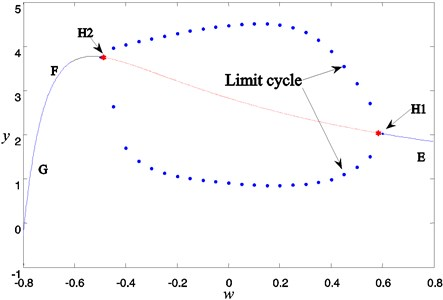Fig. 2Limit cycle of the FS Eq. (4) for w=0: a) phase portrait, b) time history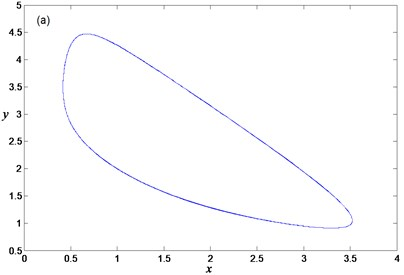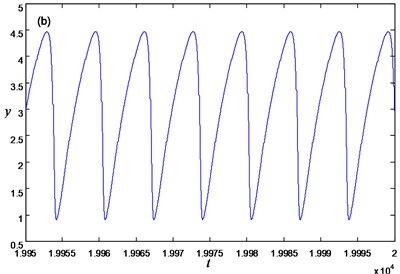### 2.2. Double-Hopf bursting oscillation and its bifurcation mechanism

Letting the frequency and amplitude of the external periodic perturbation as $\omega =$ 0.01 and $a=$ 0.7, one could obtain periodic bursting oscillation in system Eq. (2), and the phase portrait and time history are shown in Figs. 3(a) and 3(b) respectively. In each periodic oscillation, twice spiking and quiescent states exist. The frequency of the whole periodic oscillate is in accordance with perturbed frequency 0.01, while the oscillation in the spiking state may be coincide with the natural frequency of the FS Eq. (4) approximated as 0.94 (shown as Fig. 3(c)).

To further reveal the bifurcation mechanism of bursting oscillation, the transition portrait of periodic oscillation with respect to variable $y$ and slow process $w=0.7\mathrm{c}\mathrm{o}\mathrm{s}0.01t$ is shown in Fig. 4(a). Overlapping the transition portrait in Fig. 4(a) with the bifurcation diagram Fig. 1, one can obtain Fig. 4(b).

Now we can describe the evolution process of the periodic bursting oscillation in detail. Assuming that the trajectory starts from point C as shown in Fig. 4(b), one can see that the system Eq. (2) behaves with large-amplitude oscillation and is in the spiking state because of the attraction of the stable limit cycle of the FS Eq. (4). When Hopf bifurcation takes place at point $H1$, stable limit cycle disappears, and only stable focus exists in the FS Eq. (4), which leads to the oscillation amplitude decreasing gradually until the system settles down to the stable equilibrium curve. In other words, after $H1$ the spiking state gradually turns into the quiescent state with increase of $w$. When the trajectory gets to point $M$, slow variable $w=0.7\mathrm{c}\mathrm{o}\mathrm{s}0.01t$ reaches the maximum value, so that the trajectory may move with the decrease of $w$, and the system will remain the quiescent state. After passing through bifurcation point $H1$ again, the system appears gradually with small-amplitude oscillation. Along the equilibrium curve from point $D1$ to Hopf bifurcation point $H2$, the system is still in the spiking state. After that, the oscillation amplitude becomes smaller and smaller. When the stable limit cycle of the FS becomes a stable equilibrium point, the system enters the quiescent state. If $w$ approaching the minimum value, i.e. the trajectory reaches point $N$, the trajectory moves with the increase of $w$. Once again passing through the bifurcation point $H2$, the trajectory may be attracted by the stable limit cycle gradually. After passing through point $D2$, the system appears with large-amplitude oscillation and enters the spiking state again. In the whole process of the periodic bursting oscillation, it is related to two Hopf bifurcations of the FS, so that this oscillation is called as the double-Hopf periodic bursting oscillation.

Fig. 3Periodic bursting for a= 0.7: a) phase portrait, b) time history, c) partial enlargement of Fig. 3(b) in the spiking state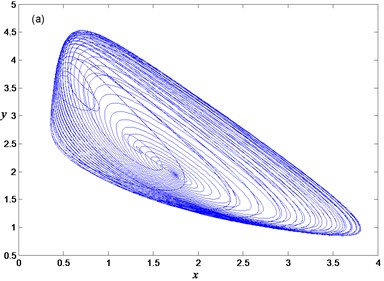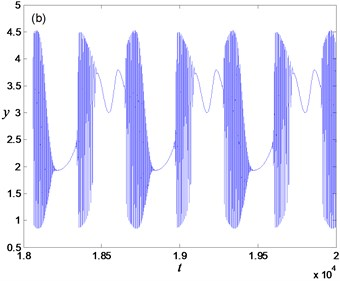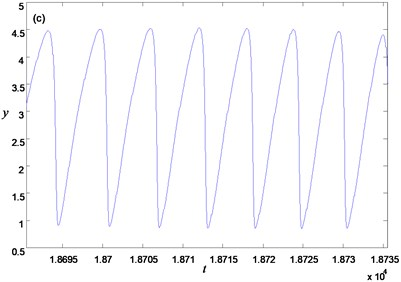Fig. 4Mechanism analysis for bursting oscillation: a) transition portrait on woy, b) overlapping of transition portrait with bifurcation diagram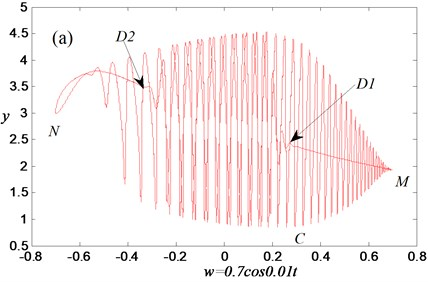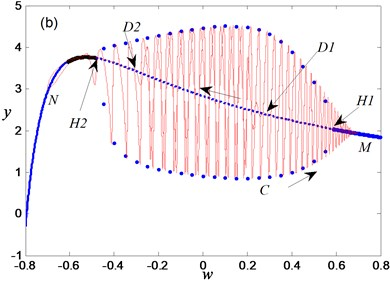Here it should be pointed out that, the whole periodic oscillation has experienced four bifurcation processes although it involves two Hopf bifurcation points of the FS. In the vicinity of each bifurcation point, there is a transition from spiking state to quiescent state, and vice versa. It is the four bifurcation behaviors that makes the double-Hopf periodic bursting oscillation in the presence of two spiking and quiescent states. In addition, when spiking and quiescent states are transferred to each other, the time-delayed behaviors may occur in the system response due to the influence of inertia and other factors. For example, when the parameter $w$ decreases gradually through the bifurcation point $H1$, the system does not immediately appear large-amplitude oscillation. After some time, it will appear large-amplitude oscillation gradually and enter the spiking state. Similarly, when the parameter $w$ increases gradually through the bifurcation point $H2$, the system also appears a Hopf bifurcation delay [11, 36, 37].

### 2.3. Effects of external periodic perturbation amplitude on bursting oscillations

As the bifurcation diagram shown in Fig. 1, two Hopf bifurcations take place at $H1$ with ${w}_{H1}=\text{0.5871}$ and $H2$ with ${w}_{H2}=$ –0.48165 respectively, which indicates that the FS Eq. (4) may possess stable limit cycle attractors for ${w}_{H2}, or stable focus attractors for $w<{w}_{H2}$ and $w>{w}_{H1}$. Thus, the change of the perturbation amplitude will make the system involves different attractors of the FS Eq. (4).

When perturbation amplitude is $a>\text{0.5871}$, the range of parameter $w$ will contain the interval [–0.5871, 0.5871], so that the trajectory of the system Eq. (2) may involve three kinds of attractors, i.e. the stable limit cycle in the middle and the stable focuses on both sides. Bilateral bi-stability of the attractors results into the four times transformations of system Eq. (2) between spiking and quiescent states. Therefore, double-Hopf bursting oscillation may exist in system Eq. (2) with two spiking and quiescent states.

When perturbation amplitude $\text{0.48165} 0.5871, the trajectory of the system only involves two kinds of attractors of FS Eq. (4), i.e. the stable focus on left side and the stable limit cycle in the middle. Accordingly, only unilateral bi-stability exists in the system, which means right bi-stability disappears and double-Hopf bursting oscillation will not occur. The time history diagram is shown in Fig. 5(a) for $a=\text{0.55}$. Compared with Fig. 3(b), it can be found that the disappearance of bi-stability on the right leads to the disappearance of the quiescent state on the right, which makes two spiking states be connected together and become into single spiking state. The single-Hopf bursting oscillation is determined by Hopf bifurcation on the left.

Fig. 5Time history diagram for: a) a= 0.55, b) a= 0.45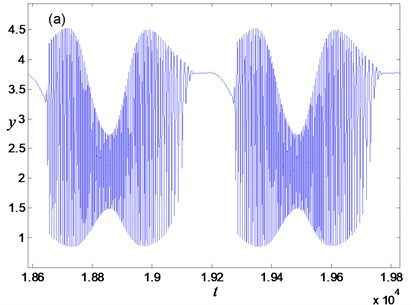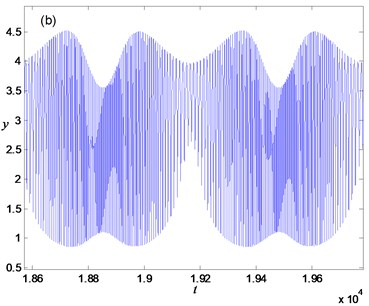For perturbation amplitude $a<\text{0.48165}$, the trajectory only involves limit cycle attractors of FS Eq. (4), which leads the system Eq. (2) to keep in spiking state without quiescent state. For example, the quasi-periodic oscillation just with large-amplitude oscillation for $a=\text{0.45}$ is plotted in Fig. 5(b).

Therefore, when the slow process of a system with two time scales is forced by external periodic perturbation, the perturbation amplitude may adjust the oscillation patterns of the system. The mechanism is that the types of attractors involved in the FS will be changed if the perturbation amplitude varies.

## 3. Bursting oscillation and bifurcation analysis in the fractional-order Brusselator

Now, we consider the following fractional-order Brusselator :

5
$\left\{\begin{array}{l}{}_{0}{}^{C}{D}_{t}^{\alpha }x\left(t\right)=A-\left(B+1\right)x+{x}^{2}y,\\ {}_{0}{}^{C}{D}_{t}^{\alpha }y\left(t\right)=Bx-{x}^{2}y,\end{array}\right\$

where $x$ and $y$ denote dimensionless concentrations of activator and inhibitor respectively. $A>0$ and $B>0$ are external parameters. ${}_{0}{}^{C}{D}_{t}^{\alpha }x\left(t\right)$ is the $\alpha$-order derivative of $x\left(t\right)$ to $t$. Here we adopt Caputo’s definition :

${}_{0}{}^{C}{D}_{t}^{\alpha }f\left(t\right)=\frac{1}{\mathrm{\Gamma }\left(n-\alpha \right)}{\int }_{0}^{t}\frac{{f}^{\left(n\right)}\left(\tau \right)}{\left(t-\tau {\right)}^{\alpha +1-n}}d\tau ,$

where $n-1<\alpha and $n$ is positive integer. When $\alpha =\text{1}$, the system Eq. (5) corresponds to the integer-order system Eq. (1).

For the equilibrium point $\left(A,B/A\right)$ of the system Eq. (5), the Jacobian matrix is:

$J=\left(\begin{array}{cc}A-1& {A}^{2}\\ -B& -{A}^{2}\end{array}\right),$

and the corresponding characteristic values can be expressed as ${\lambda }_{1,2}=\frac{1}{2}\left(trJ±\sqrt{t{r}^{2}J-4\mathrm{d}\mathrm{e}\mathrm{t}J}\right)$, where $trJ=B-1-{A}^{2}$, $\mathrm{d}\mathrm{e}\mathrm{t}J={A}^{2}$.

It is well-known for the integer-order system Eq. (1) that a steady-state solution becomes unstable according to the Hopf bifurcations $B>1+{A}^{2}$, ${A}^{2}>0$.

For $0<\alpha <2$, the value $\alpha$ is regarded as a bifurcation parameter. When $t{r}^{2}J<4\mathrm{d}\mathrm{e}\mathrm{t}J$, we can introduce a marginal value ${\alpha }_{0}=\frac{2}{\pi }\left|\mathrm{a}\mathrm{r}\mathrm{g}\left({\lambda }_{i}\right)\right|$, $\left(i=$ 1, 2$\right)$, which could be formulated as:

6
${\alpha }_{0}=\left\{\begin{array}{ll}\frac{2}{\pi }\mathrm{a}\mathrm{r}\mathrm{c}\mathrm{t}\mathrm{a}\mathrm{n}\sqrt{\frac{4\mathrm{d}\mathrm{e}\mathrm{t}J}{t{r}^{2}J}-1},& trJ>0,\\ 2-\frac{2}{\pi }\mathrm{a}\mathrm{r}\mathrm{c}\mathrm{t}\mathrm{a}\mathrm{n}\sqrt{\frac{4\mathrm{d}\mathrm{e}\mathrm{t}J}{t{r}^{2}J}-1},& trJ<0.\end{array}\right\$

External periodic perturbation factor is still considered, and the dynamic model is written as:

7
$\left\{\begin{array}{l}{}_{0}{}^{C}{D}_{t}^{\alpha }x\left(t\right)=A-\left(B+1\right)x+{x}^{2}y,\\ {}_{0}{}^{C}{D}_{t}^{\alpha }y\left(t\right)=Bx-{x}^{2}y+a\mathrm{c}\mathrm{o}\mathrm{s}\omega t,\end{array}\right\$

where $a$ is external periodic perturbation amplitude and $\omega$ is external periodic perturbation frequency.

### 3.1. Bifurcation analysis

Letting $\theta =\omega t$, Eq. (7) can be converted into an autonomous form:

8
$\left\{\begin{array}{l}{}_{0}{}^{C}{D}_{t}^{\alpha }x\left(t\right)=A-\left(B+1\right)x+{x}^{2}y,\\ \begin{array}{l}{}_{0}{}^{C}{D}_{t}^{\alpha }y\left(t\right)=Bx-{x}^{2}y+a\mathrm{c}\mathrm{o}\mathrm{s}\theta ,\\ \frac{d\theta }{dt}=\omega .\end{array}\end{array}\right\$

If the perturbation frequency is much less than the natural frequency of the original system at least one order of magnitude, the reaction is a coupling system of two time scales. By defining $w=a\mathrm{c}\mathrm{o}\mathrm{s}\theta$, the FS can be written as:

9
$\left\{\begin{array}{l}{}_{0}{}^{C}{D}_{t}^{\alpha }x\left(t\right)=A-\left(B+1\right)x+{x}^{2}y,\\ {}_{0}{}^{C}{D}_{t}^{\alpha }y\left(t\right)=Bx-{x}^{2}y+w.\end{array}\right\$

For the abovementioned fixed parameter $A=\text{1.06}$ and $B=\text{3}$, the critical value of the stability region of the FS Eq. (9) will also be changed when the slow variable parameter $w$ varies. For the equilibrium point ${E}_{0}\left(A+w,\left(AB+wB+w\right)/\left(A+w{\right)}^{2}\right)$ of the FS Eq. (9), the corresponding characteristic equation reads ${\lambda }^{2}+\left(1-B+\left(A+w{\right)}^{2}-2w/\left(A+w\right)\right)\lambda +\left(A+w{\right)}^{2}=0$.

Combined Eq. (6), the double-parameter bifurcation diagram of FS Eq. (9) with respect to the parameters $w$ and the order ${\alpha }_{0}$ is plotted in Fig. 6. For $\text{0.69282}<\alpha <\text{2}$, two Hopf bifurcation points exist in the FS Eq. (9). With the decrease of $\alpha$, the distance between the two critical pints of Hopf bifurcation is getting smaller and smaller. In the end, the two points “collide” and disappear. For $0<\alpha <\text{0.6928}$, the Hopf bifurcation does not occur for the FS Eq. (9).

Fig. 6The bifurcation diagram of FS Eq. (9) with respect to parameters w and α0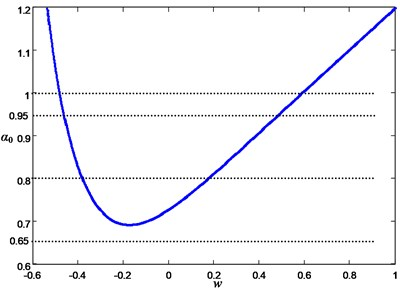### 3.2. Effects of fractional order on bursting oscillations

As shown in Fig. 6, there are two Hopf bifurcation points for $\text{0.69282}<\alpha <\text{2}$ in FS Eq. (9), and the Hopf bifurcation does not occur for $\text{0}<\alpha <\text{0.6928}$. For example, at $\alpha =\text{0.95}$, $I1$ and $I2$ are two Hopf bifurcation points, and the corresponding parameter values are ${w}_{I1}=\text{0.4844}$ and ${w}_{I2}=$ –0.4624 (see Fig. 7). Similar to the integer-order system, a periodic oscillation of the fractional-order system has still twice spiking and quiescent states. The difference is that as ${\alpha }_{0}$ becomes smaller, the distance between the two Hopf bifurcation points becomes much smaller, which leads to the time during the spiking state becomes much shorter, while the time during the quiescent state becomes much longer. When $\alpha =\text{0.65}$, the Hopf bifurcation point disappears. Although the system is still doing periodic motion, but there is no bursting phenomenon occurs (see Fig. 8). Therefore, the fractional order $\alpha$ plays an important role to control the bursting oscillation of the system.

For numerical simulations, the fractional-order differential system is different from integer-order one [38-41]. Here the Power Series Expansion method  is applied to compute the numerical solution of the fractional-order Brusselator. The phase portraits and time histories in Fig. 7 and Fig. 8 are plotted by MATLAB. Here the total computation time is 20000 s, and the step length is 0.001 s.

Fig. 7Periodic oscillation for α=0.95,a=0.7: a) phase portrait, b) time history, c) transition portrait on woy plane, d) overlapping of transition portrait with bifurcation diagram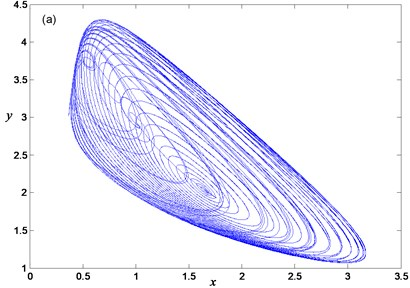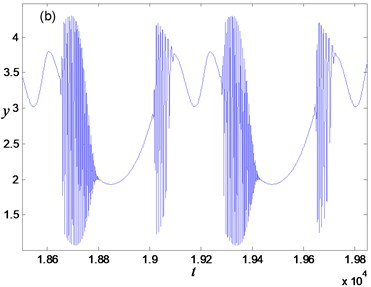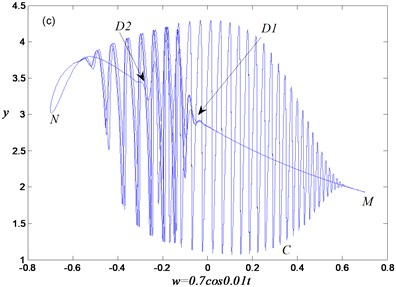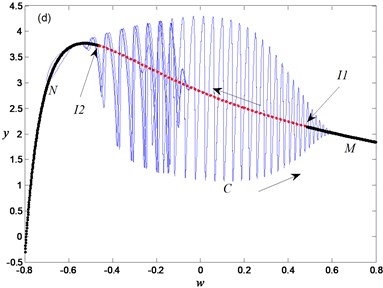Fig. 8Periodic oscillation for α=0.65, a=0.7: a) phase portrait, b) time history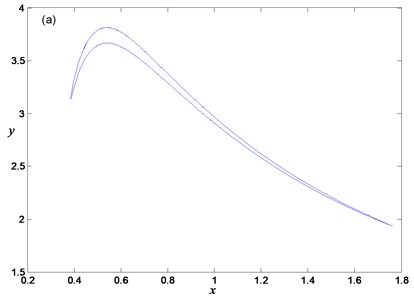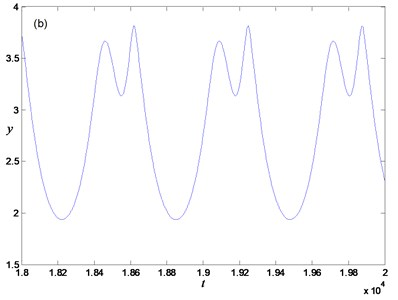## 4. Conclusions

In this paper, the integer-order and fractional-order Brusselators with external periodic perturbation are studied. When the external periodic disturbance frequency is much smaller than the system natural frequency, the system has two different time scales and shows an obvious slow-fast effect. The typical bursting behaviors such as double-Hopf and single-Hopf bursting oscillations are found, and the slow-fast analysis method is used to reveal generation mechanism of bursting oscillation about Brusselator. In fact, the Hopf bifurcation of FS leads to transitions between spiking and quiescent states. And the transition times of spiking and quiescent states in one period are decided by the numbers of critical points of Hopf bifurcation.

The influences of different parameters on bursting phenomenon are discussed in details. When the amplitude of periodic disturbance is larger, the system involves two Hopf bifurcation points of FS and three kinds of attractors. The bilateral bi-stability of attractors results into four times transitions between spiking and quiescent states, i.e. double-Hopf bursting oscillation occurs. With the decrease of amplitude, the system involves one Hopf bifurcation point of FS and two kinds of attractors. The unilateral bi-stability of attractors results into twice transitions between spiking and quiescent states, i.e. single-Hopf bursting oscillation appears. When the amplitude continues to decrease, the system no longer involves the critical point of Hopf bifurcation, and only relates to limit cycle attractors. The single stability leads the system to keep in spiking state without quiescent state, but the whole periodic motion is simple oscillation with two frequencies.

In addition, the fractional order plays an important role to control the bursting behaviors of the system. With the decrease of fractional order, the number of Hopf bifurcation points becomes from two to one, and finally zero. Correspondingly, the system response is changed from periodic bursting oscillation to simple period oscillation, that means the phenomenon of busting disappears. The results may provide important theoretical basis for the further study of Brusselator model.

#### Cited by

Effect of geometry configuration on bursting oscillations of the mechanical oscillator with strong irrational nonlinearities and its FPGA-based implementation
Bursting, mixed-mode oscillations and homoclinic bifurcation in a parametrically and self-excited mixed Rayleigh–Liénard oscillator with asymmetric double well potential

17 December 2016
Accepted
16 February 2017
Published
31 March 2017
SUBJECTS
Fractional dynamics and applications
Keywords
fractional-order system
Brusselator
bursting oscillation
Hopf bifurcation
Acknowledgements

The authors are grateful to the support from the National Natural Science Foundation of China (Grant Nos. 11372196 and 11672191), Natural Science Foundation of Hebei Province (Grant No. A2014210062 and A2014210104), and the Training Program for Leading Talent in University Innovative Research Team in Hebei Province (LJRC006).